# HSPT Math : Concepts

## Example Questions

### Example Question #41 : Negative Numbers

Whch of the following is the least of the four expressions?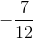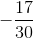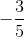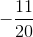Explanation:

Rewrite each of the numbers with a common denominator. To find that common denominator, examine the prime factorizations of 5, 12, 20, and 30: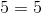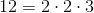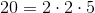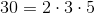Multiply: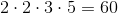Rewrite each fraction with deniominator 60: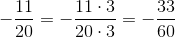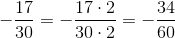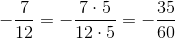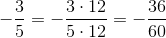Of these four negative numbers,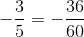has the greatest absolute value, so it is the least of the four, and the correct choice.

### Example Question #1 : Understand An Inequality On A Number Line: Ccss.Math.Content.6.Ns.C.7a

Fill in the blank with the proper sign.__________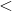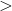Explanation:

In order to solve this problem, we will use a number line. Numbers to the left of zero on the line are less than numbers to the right.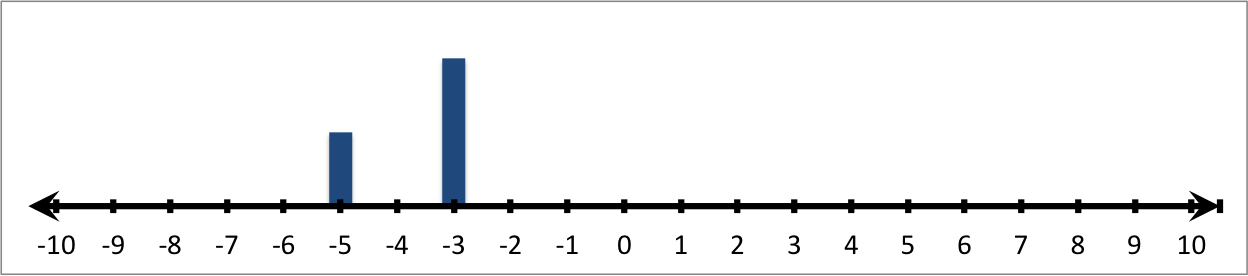Therefore: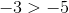### Example Question #41 : Concepts

Fill in the blank with the proper sign.__________Explanation:

In order to solve this problem, we will use a number line. Numbers to the left of zero on the line are less than numbers to the right.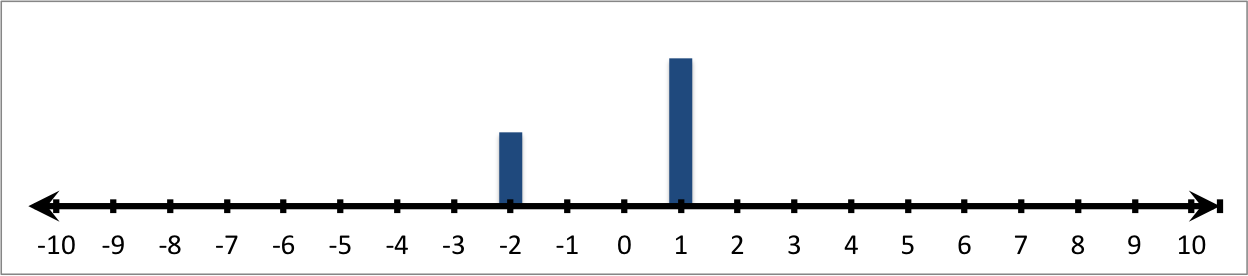Therefore: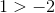### Example Question #1 : Understand An Inequality On A Number Line: Ccss.Math.Content.6.Ns.C.7a

Fill in the blank with the proper sign.__________Explanation:

In order to solve this problem, we will use a number line. Numbers to the left of zero on the line are less than numbers to the right.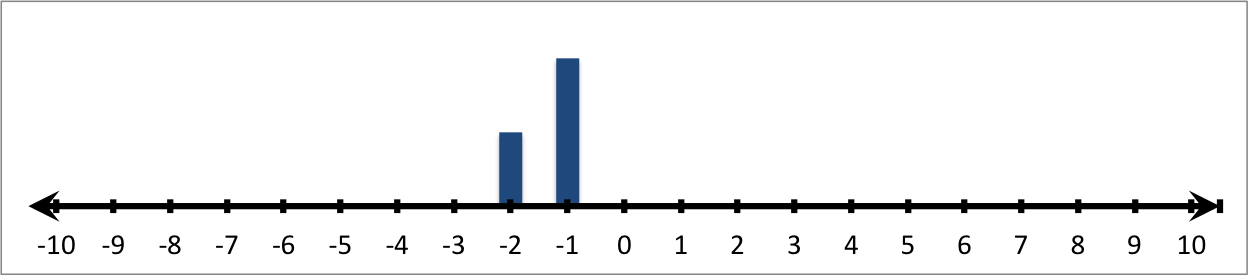Therefore: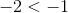### Example Question #42 : Concepts

Fill in the blank with the proper sign.__________Explanation:

In order to solve this problem, we will use a number line. Numbers to the left of zero on the line are less than numbers to the right.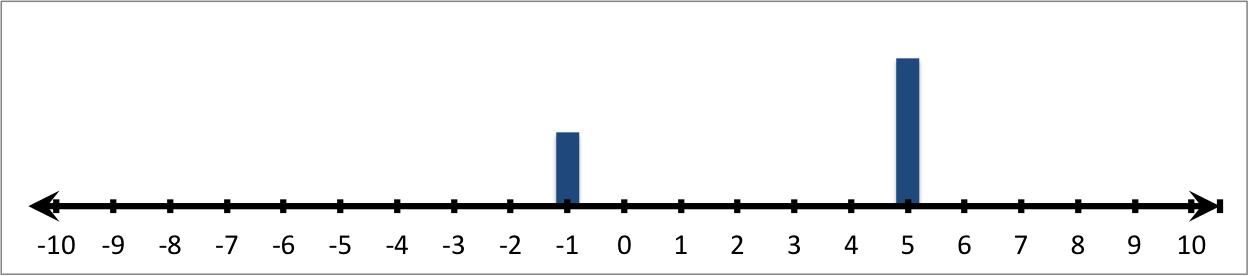Therefore: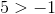### Example Question #451 : The Number System

Fill in the blank with the proper sign.__________Explanation:

In order to solve this problem, we will use a number line. Numbers to the left of zero on the line are less than numbers to the right.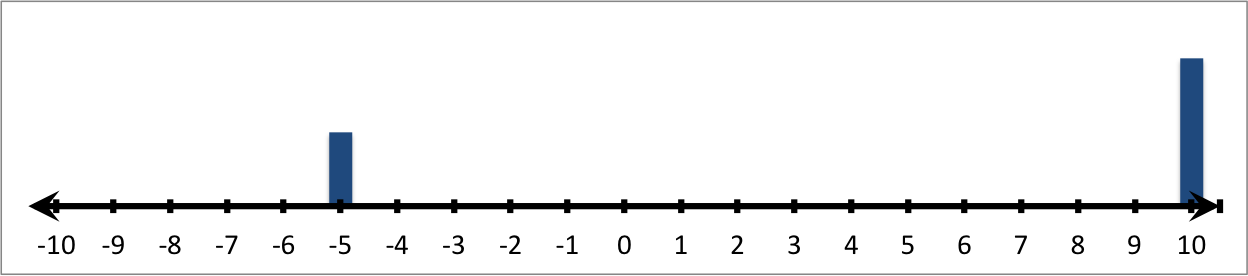Therefore: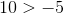### Example Question #1 : Understand An Inequality On A Number Line: Ccss.Math.Content.6.Ns.C.7a

Fill in the blank with the proper sign.__________Explanation:

In order to solve this problem, we will use a number line. Numbers to the left of zero on the line are less than numbers to the right.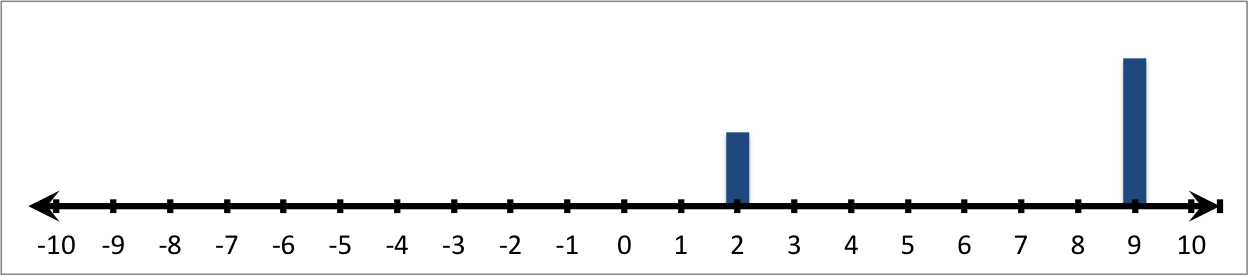Therefore: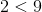### Example Question #1 : Understand An Inequality On A Number Line: Ccss.Math.Content.6.Ns.C.7a

Fill in the blank with the proper sign.__________Explanation:

In order to solve this problem, we will use a number line. Numbers to the left of zero on the line are less than numbers to the right.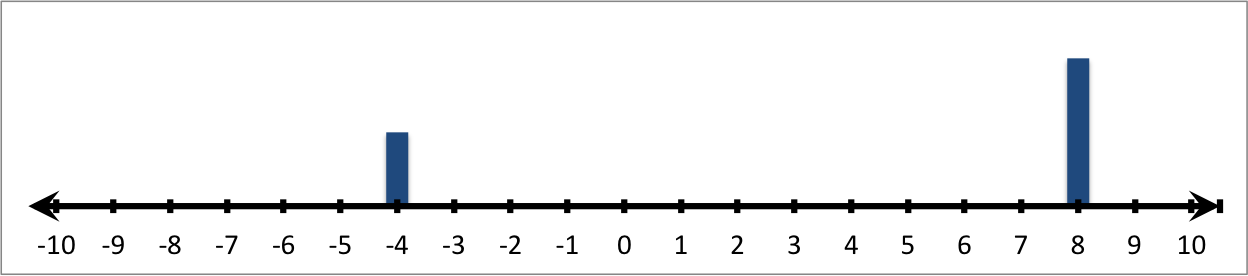Therefore: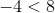### Example Question #1 : Understand An Inequality On A Number Line: Ccss.Math.Content.6.Ns.C.7a

Fill in the blank with the proper sign.__________Explanation:

In order to solve this problem, we will use a number line. Numbers to the left of zero on the line are less than numbers to the right.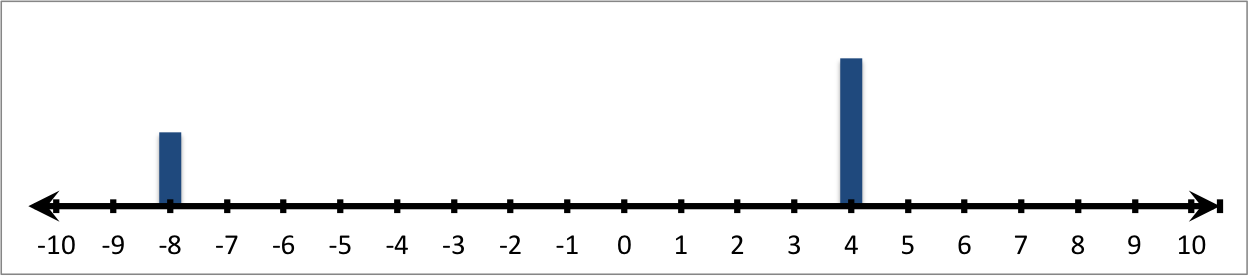Therefore: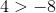### Example Question #1 : Understand An Inequality On A Number Line: Ccss.Math.Content.6.Ns.C.7a

Fill in the blank with the proper sign.__________Explanation:

In order to solve this problem, we will use a number line. Numbers to the left of zero on the line are less than numbers to the right.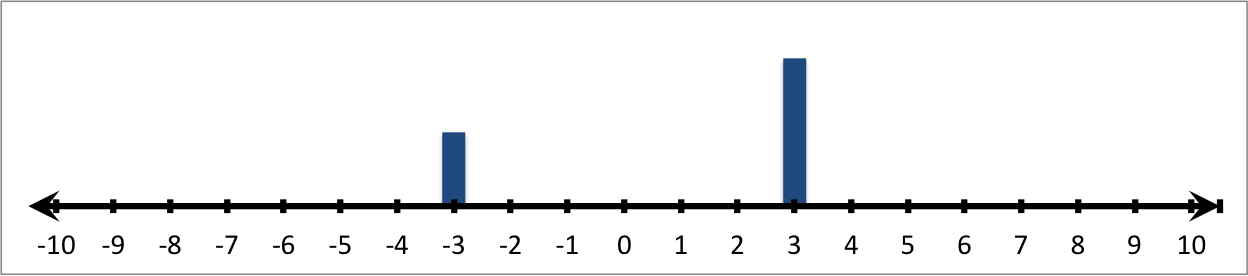Therefore: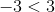### All HSPT Math Resources